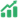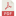# EXPLORING STUDENTS' COGNITIVE PROCESSES IN SOLVING NUMERACY TASK OF GEOMETRY AND MEASUREMENT REVIEWED BY MATHEMATICAL ABILITY

• Citra Cahyaning Pertiwi Universitas Negeri Surabaya
• Rooselyna Ekawati

### Abstract

This study aims to analyze how the cognitive processes of high school students in terms of their mathematical abilities in solving the Minimum Competency Assessment Numeracy problems related to Geometry and Measurement. Problems were given to three 10th science students with categories of high, medium, and low mathematical ability. Data on the results of the subject's work were analyzed based on the cognitive processes stage, namely the stage of Comprehending linguistic and numerical information in the problem; 2)  Translating and transforming that information into mathematical notations, algorithms, and equations; 3) Observing relationships among the elements of the problem; 4) Formulating a plan to solve the problem; 5) Predicting outcome; 6) Regulating the solution path as it is executed; 7) Detecting and correcting errors during problem solution which is then followed by in-depth interviews. The results showed that students with high mathematical abilities went through all stages of the cognitive process quite coherently and completely. While students with moderate abilities went through the stage of understanding information to the calculation stage of planning, but there were some errors and corrections in finding solutions. Meanwhile, students with low abilities had not been able to show the stages of cognitive processes in a coherent manner so that many stages were interrupted and had to return to the stage of understanding the problem. Based on these results, it is hoped that learning for the preparation of the Minimum Competency Assessment for Geometry and Measurement material can be emphasized more and so that the understanding of information on students' problems can continue the completion stage according to the solution plan that they have made.

Keywords: Cognitive Processes, Numeracy, Geometry and Measurement.

Published
2022-01-26
Section
ArticlesAbstract View: 76PDF_181-197 Download: 58